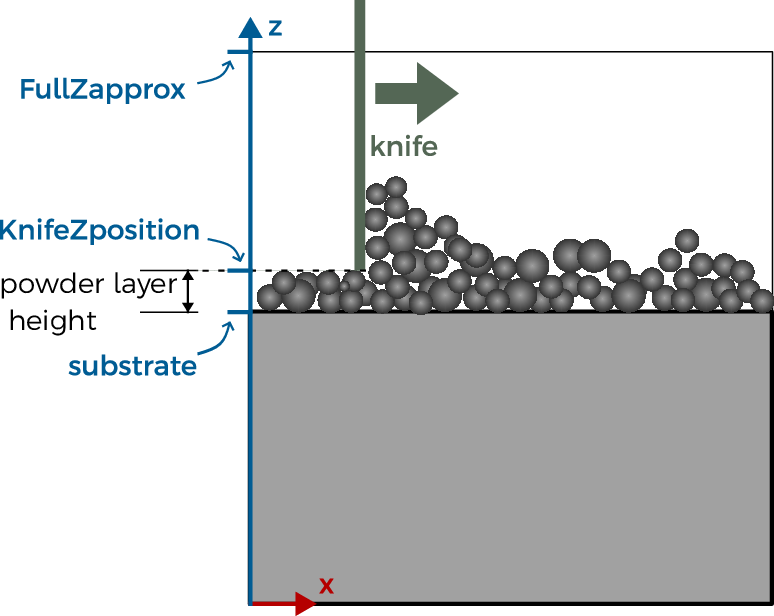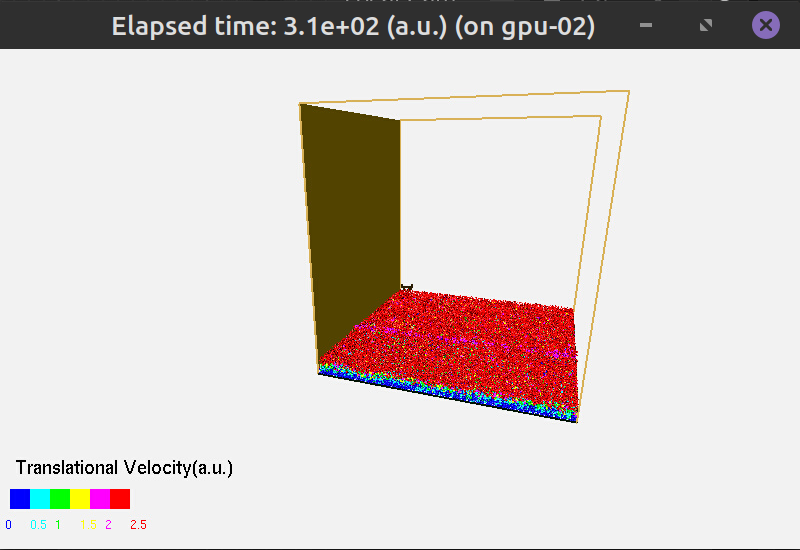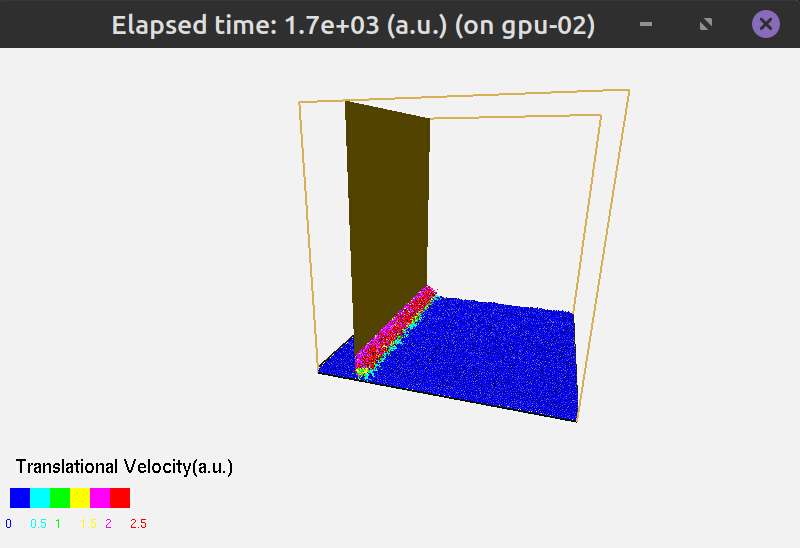# Powder Generation¶

## Powder Simulation¶

The powder generation software runs simulation of powder deposition. Then a knife moves along x axis at the specified height.

The parameters are set in the same JSON file that is used in simulation. This helps with the consistency of simulation sizes.

## Input Configuration¶

To start a simulation with internally generated powder layer, prepare a JSON file for your simulation as usual, but include the powder generation setting. As usual, if the setting is not included, the default value is used.

First, look at the Sizes and PowderBedGenerator sections of the input JSON file. Here is the example

"sizes": {
"FullXapprox": 0.010,
"FullYapprox": 0.010,
"FullZapprox": 0.005,
"substrate"  : 0.00456
},
"PowderBedGenerator": {
"Type": "PowDEM",
"RandomSeed": 0,
"GroundSurface": "",
"PSDsizes":
[[0, 45e-6], [5, 50e-6], [30, 60e-6], [50,70e-6], [70,80e-6], [90,90e-6], [100,100e-6]],
"KnifeZposition": 0.00466
},


Here are the solver options.

Key

Value Type

Value

Type

string

PowDEM

The name of the powder generator software.

RandomSeed

integer

0

To generate different configurations of powder with same parameters, change this number to any other number.

KnifeZposition

float

0.00466

The height of the powder spreading knife (m).

GroundSurface

string

“”

Leave empty for powder deposition on a flat surface, specified with the sizes.substrate parameter in JSON. Fill in the file path to the surface geometry if the substrate is not flat.

PSDsizes

list of value pairs

[[0, 45e-6], [5, 50e-6],…}

The distribution of powder sizes is set in the form $$[[p_1,d_1],[p_2,d_2], ...]$$, where $$p_i$$ is the volume fraction of powder particles which have diameter less than $$d_i$$. The pairs should be listed in order of increasing diameter.

Here are the geometry options.

Key

Value Type

Value

FullXapprox

float

0.010

FullYapprox

float

0.010

FullZapprox

float

0.005

substrate

float

0.00456

The FullXapprox$$\times$$ FullYapprox (Here, 10 mm $$\times$$ 10 mm) area is filled with powder. The height of the powder layer has to be (much) less than FullZapprox (m). The powder is set on the substrate, the height of the substrate is substrate (m). These are the same fields that are used in the KiSSAM simulation. The height of the powder layer is KnifeZposition-substrate (here, 0.1 mm).

$$0<$$ substrate $$<$$ KnifeZposition $$<$$ FullZapprox.## PowDEM run¶

Launch the powder deposition simulation with :

/share/bin/PowDEM [<default.json>] <input.json> [-n] [-v]


If the fields which are required by the program are absent from the input.json file, they can be provided in the default file default.json

-v

verbose output.

-n

turn off interactrive graphics.## Output Files¶

• spheres.dat contains the complete data of the generated powder geometry.

This is a text file and it contains four columns. For each sphere, its position ($$x$$, $$y$$, $$z$$) and radius ($$r$$) in μm are in one row.

27.4911 27.4917 27.4911 27.5
44.7133 79.1247 63.0167 37.5
87.07 32.4956 32.4953 32.5
166.9 34.6008 27.4979 27.5
128.672 75.4377 27.498 27.5
...


Before including the generated script in the KiSSAM simulation, spheres have to be rasterized with the rasterizeSpheres.x script.

• powder_evolution.log contains the detailed info of the particles dynamics and its relaxation to a steady-state solution.

It is a file in text format with several columns. Rows starting wit # are comments. Check this file during the simulation to check the integral values of the current powder geometry.

Example:

# Powder simulation convergence:
# t_sim Xmean Ymean Zmean Ymin Ymax Vmean V_Ymax V_0.99N maxV_traj maxV_insta Num_Particles
0 497.62 349.225 501.327 0 582.428 1 1 1 1 1 2098
...
1957.13 1234.56 494.681 41.2317 499.222 0 100.166 0.00786938 -0.0049597 0.0404546 0.0800193 0.147372 1084


The columns contain:

Column Key

Description

t_sim

time in seconds

X

Knife position in $$x$$ coordinate

Xmean

mean $$x$$ position of particles

Ymean

mean $$y$$ position of particles

Zmean

mean $$z$$ position of particles

Ymin

minimal $$y$$ coordinate ($$y$$ is the vertical axis)

Ymax

maximal $$y$$ coordinate ($$y$$ is the vertical axis)

Vmean

mean velocity of particles

V_Ymax

velocity of max height change

V_0.99N

velocity of 99% fastest particle

maxV_traj

maximum trajectory velocity ($$\Delta x/\Delta t$$ for a given time step Delta t)

maxV_insta

maximum instantaneous velocity

Num_Particles

number of spheres in the simulation

• powder_stats.log is a text log file which contains statistic information of the powder.

It might be useful in case of further powder analysis. This file contains the final thickness of the powder (the difference between the highest and lowest point of the powder by the vertical axis), the integral filling factor. It is followed by a table of three columns: particle size, distribution density by volume, distribution density by total amount, respectively. The file header is as follows:

# Final characteristics of the powder:
# Powder thickness (max) = 119.23 um, filling factor (mean) = 50.8124%. For more details please use spheres-analyze utility.
# PSD statistics:
# size_i(um) fi_volumetric fi_number


## Use Powder in the Simulation¶

To use the prepared powder in the simulation, prepare the volumetric data from the spheres.dat file. To do this, use the rasterizeSpheres.x script. For example,

/opt/KiSSAM/scripts/rasterizeSpheres.x 3 spheres.dat


Here, 3 is the mesh step in μm. The same mesh step should be used in the KiSSAM simulation in the NumericalParams.dr field (see JSON file description).

The file that is generated by the script should be specified as initial powder geometry is the simulation.

"Powder": {
"initSpheresVDBfile": "init_spheres.vdb"
}


## Visualization controls¶

The visualization is interactive. The following keyboard and mouse actions control the run.

• Space is to pause the simulation. Press Space again to resume the run.

• Press w/e is to zoom in/out the picture.

• Mouse buttons and actions

• Press the middle mouse button (wheel) and move mouse to translate the picture.

• Press the left mouse button and move the mouse to rotate the picture.